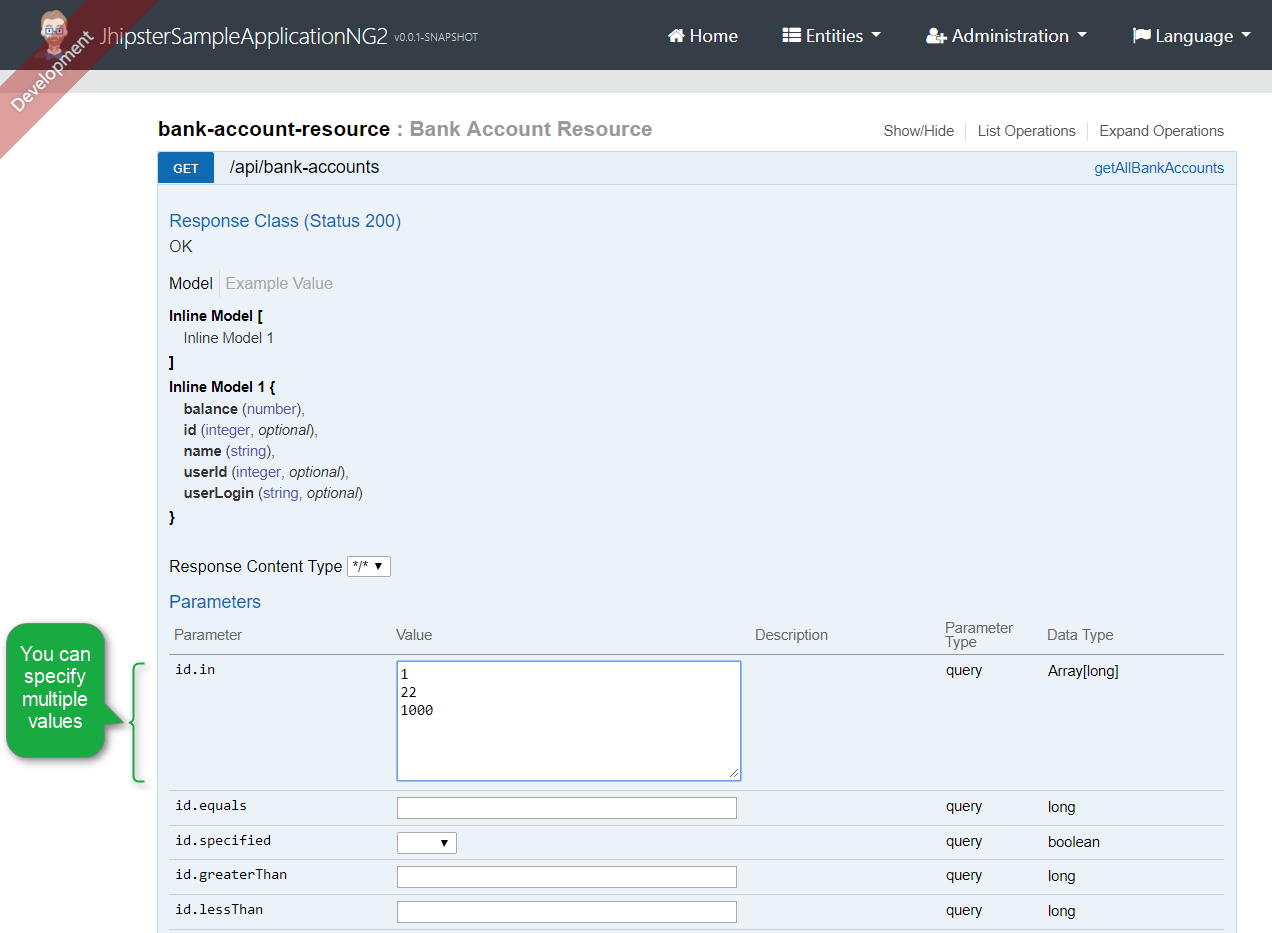过滤您的实体类

公共接口

• For each xyz field
• xyz.equals=someValue
• To list all the entities, where xyz equals to ‘someValue’
• xyz.in=someValue,otherValue
• To list all the entities, where xyz equals to ‘someValue’ or ‘otherValue’
• xyz.specified=true
• To list all the entities, where xyz is not null, specified.
• xyz.specified=false
• To list all the entities, where xyz is null, unspecified.
• If xyz’s type is string:
• xyz.contains=something
• To list all the entities, where xyz contains ‘something’.
• If xyz’s is either any of the number types, or the date types.
• xyz.greaterThan=someValue
• To list all the entities, where xyz is greater than ‘someValue’.
• xyz.lessThan=someValue
• To list all the entities, where xyz is less than ‘someValue’.
• xyz.greaterOrEqualThan=someValue
• To list all the entities, where xyz is greater than or equal to ‘someValue’.
• xyz.lessOrEqualThan=someValue
• To list all the entities, where xyz is less than or equal to ‘someValue’.实现

EntityQueryService, 我们将Criteria对象转换为静态的、类型安全的JPA查询对象。为此, 它 需要 在构建过程中设置static metamodel generation 为 enabled. 详见 JPA静态元模型生成器文档 for details.